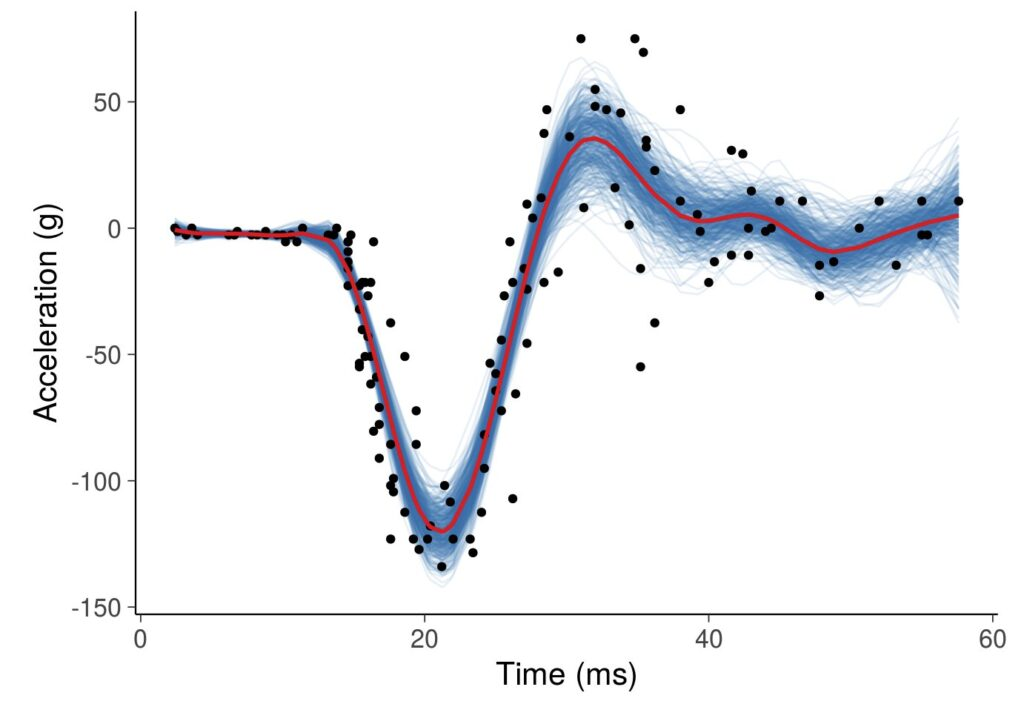# 190.012 Introduction to Machine Learning (2SH L, SS)This course is based on the Machine Learning book by Univ.-Prof. Dr. Elmar Rueckert.

It is written for experienced undergraduates or for first

The lecture provides the basic knowledge for the application of modern machine learning methods. It includes an introduction to the basics of data modeling and probability theory. Classical probabilistic linear and non-linear regression methods are derived and discussed using practical examples.

## Course Topics

1. Introduction to Machine Learning (Data and modelling fundamentals)
2. Introduction to Probability Theory (Statistics refresher, Bayes Theorem, Common Probability distributions, Gaussian Calculus).
3. Linear Probabilistic Regression (Linear models, Maximum Likelihood, Bayes & Logistic Regression).
4. Nonlinear Probabilistic Regression (Radial basis function networks, Gaussian Processes, Recent research results in Robotic Movement Primitives, Hierarchical Bayesian & Mixture Models).
5. Probabilistic Inference for Time Series (Time series data, basis function models, learning).

## Learning objectives / qualifications

• Students get a comprehensive understanding of basic probability theory concepts and methods.
• Students learn to analyze the challenges in a task and to identify promising machine learning approaches.
• Students will understand the difference between deterministic and probabilistic algorithms and can define underlying assumptions and requirements.
• Students understand and can apply advanced regression, inference and optimization techniques to real world problems.
• Students know how to analyze the models’ results, improve the model parameters and can interpret the model predictions and their relevance.
• Students understand how the basic concepts are used in current state-of-the-art research in robot movement primitive learning and in neural planning.

The course will be graded based on a written exam (100 Points). 50% of all questions need to be answered correctly to be positive. The exam will take place in the classroom, or online, depending on the current university regulations.

In addition, up to 10 bonus points obtained in regular quiz sessions in the classroom, and 20% of the achieved points of the Machine Learning Lab will be added to your exam result. Note that bonus points can only be obtained when attending the lectures in person.

Grading scheme: 0-49.9Pts (5), 50-65.9Pts (4), 66-79Pts (3), 80-91Pts (2), 92-100Pts (1).

Forthcoming exam dates are:

• 30.05.2023 at 11:00 Office of Prof. Rueckert at CPS, Metallurgie building 1st floor
• 21.06.2023 at 13:00 HS 1 Studienzentrum
• 05.07.2023 at 11:00 Office of Prof. Rueckert at CPS, Metallurgie building 1st floor
• 11.10.2023 at 13:15 TBD
• More dates upon request via email to teaching@ai-lab.science (send your request one month in advance to the desired exam date).

## Literature

• The Probabilistic Machine Learning book by Univ.-Prof. Dr. Elmar Rueckert.
• Daphne Koller, Nir Friedman. Probabilistic Graphical Models: Principles and Techniques. ISBN 978-0-262-01319-2
• Christopher M. Bishop. Pattern Recognition and Machine Learning. Springer (2006). ISBN 978-0-387-31073-2.
• David Barber. Bayesian Reasoning and Machine Learning, Cambridge University Press (2012). ISBN 978-0-521-51814-7.
• Kevin P. Murphy. Machine Learning: A Probabilistic Perspective. ISBN 978-0-262-01802-9